# y[n]-5y[n-1]+6y[n-2]=2x[n]-4x[n-1] Basic Fourier Transform

The relation between input and output is from the Fourier transform of

the system given as

Find the pulse response $$h$$ [n] using.

$$y[n]-5 y[n-1]+6 y[n-2]=2 x[n]-4 x[n-1]$$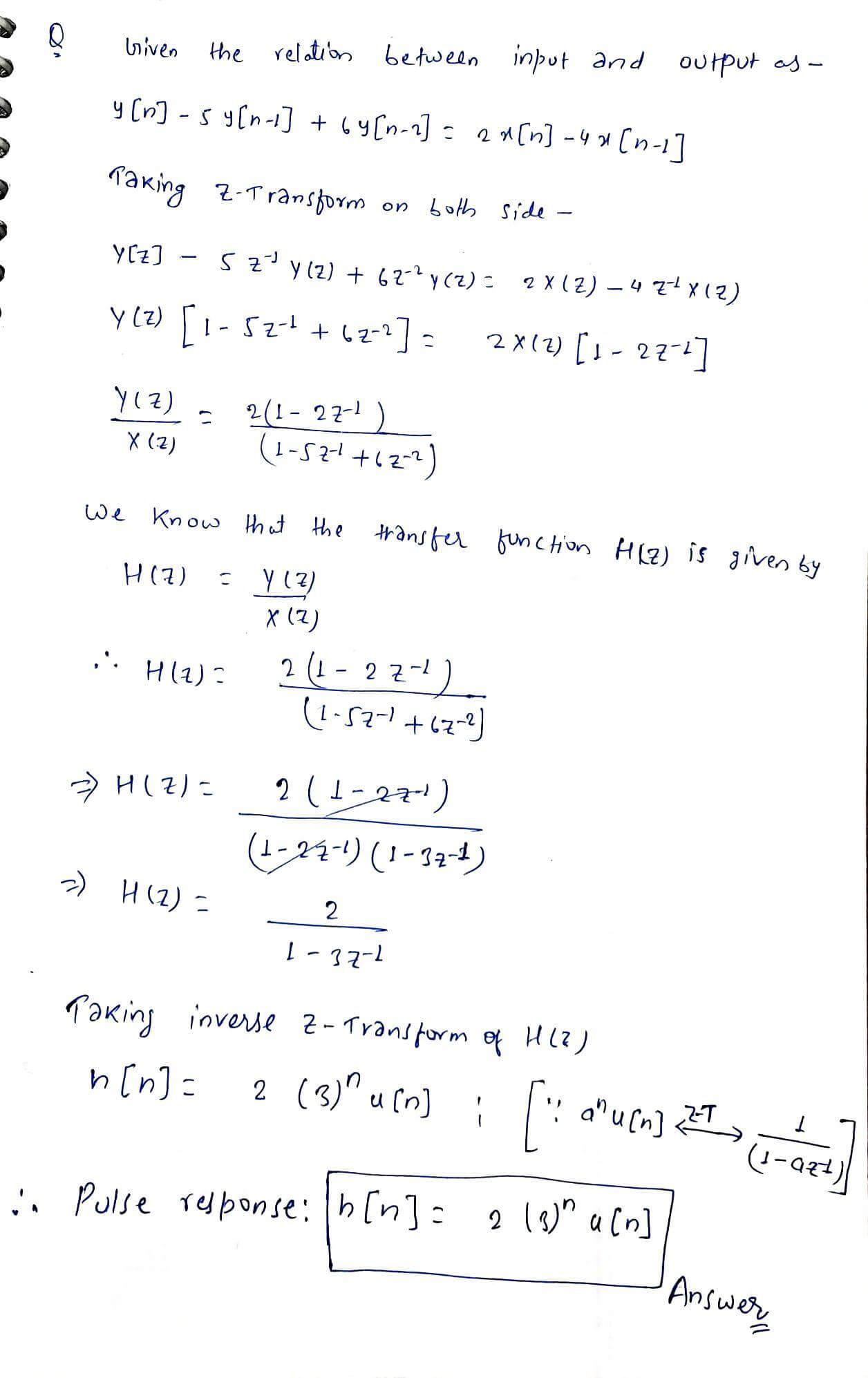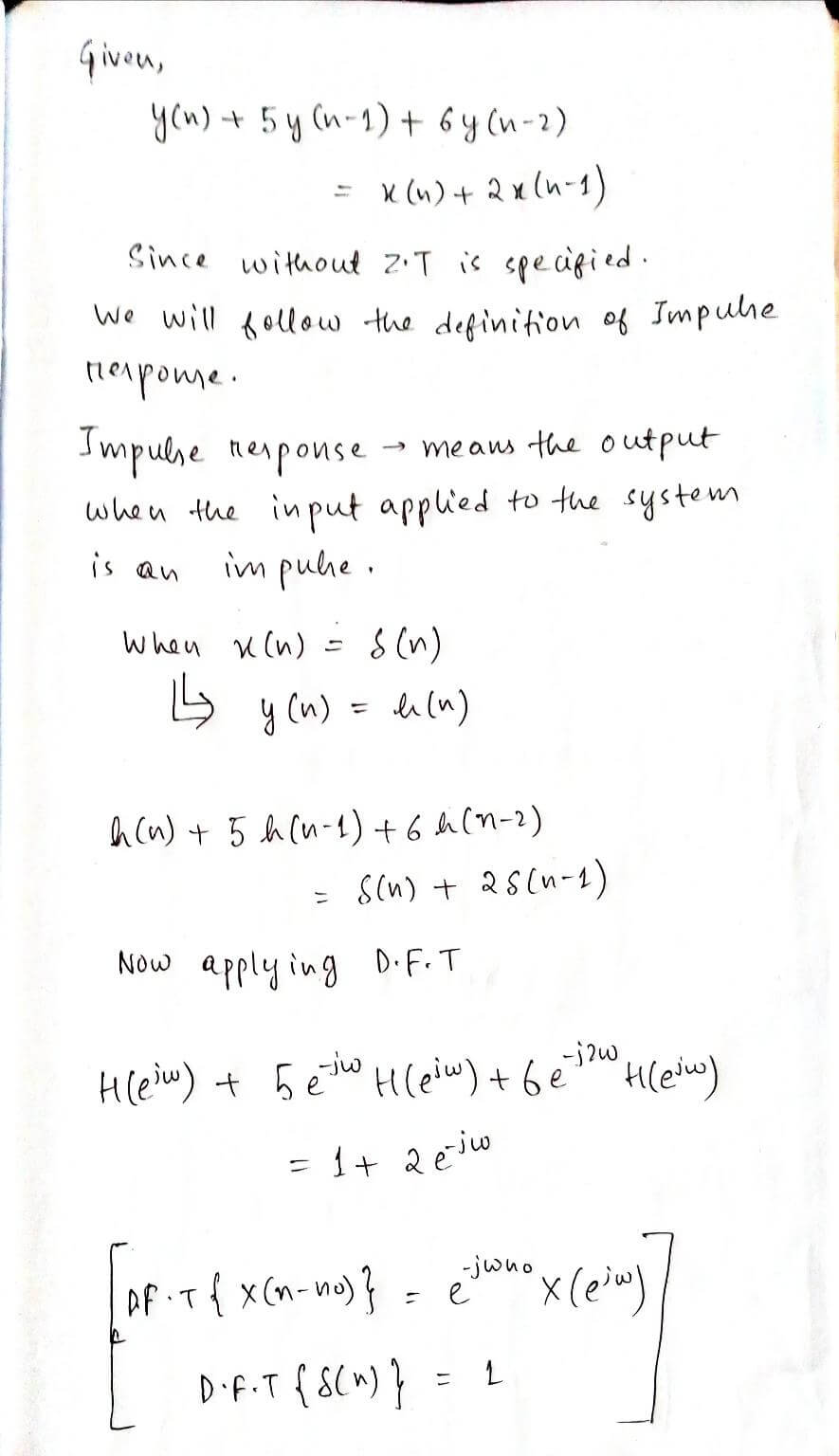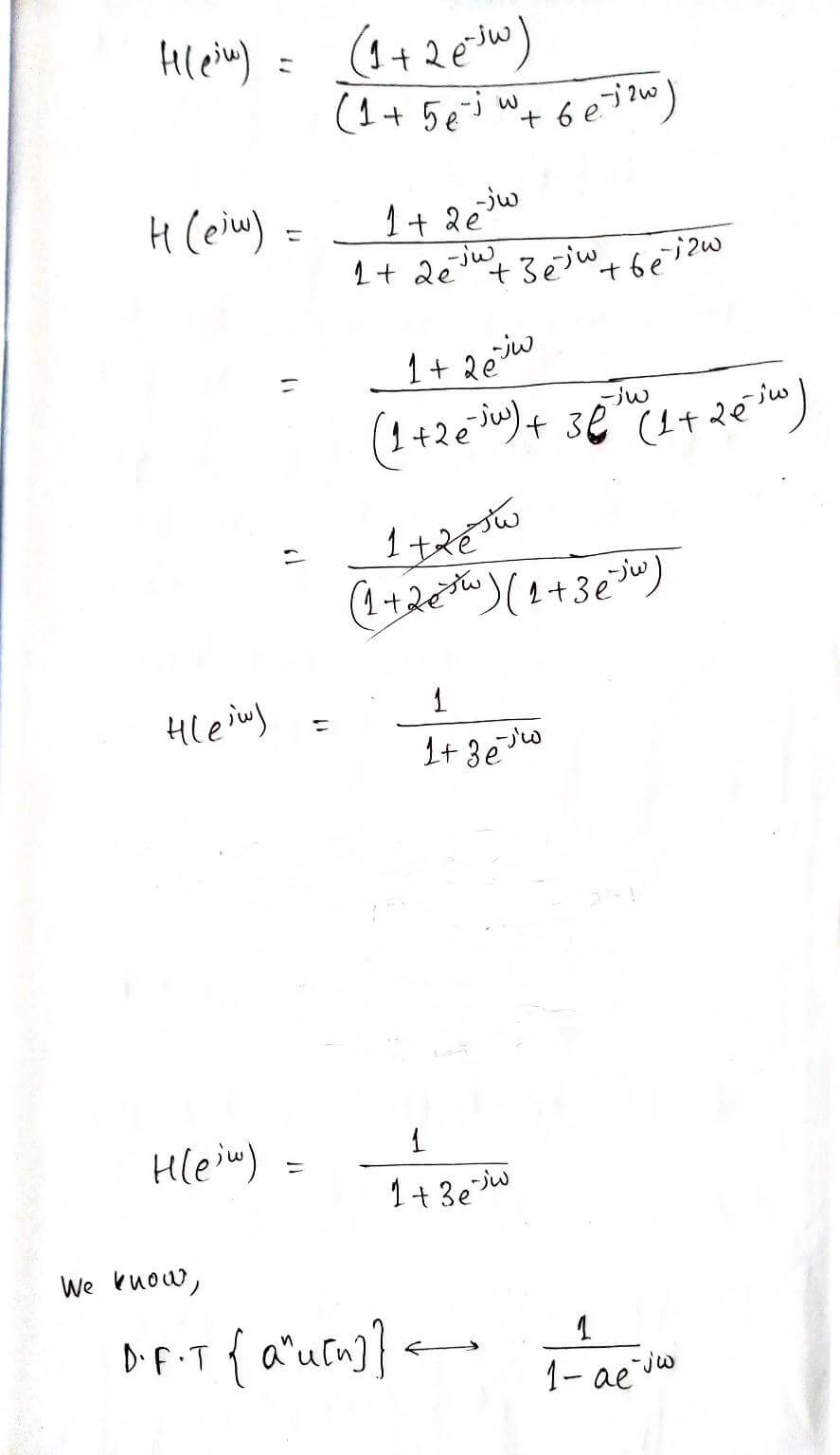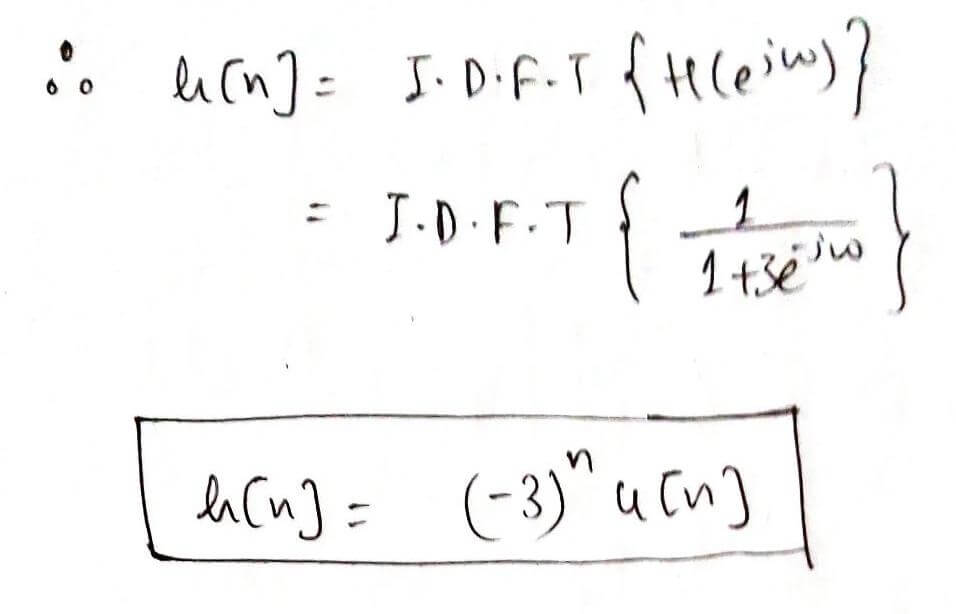#### Earn Coin

Coins can be redeemed for fabulous gifts.

Similar Homework Help Questions
• ### 1. Using appropriate properties and the table of Fourier transforms, obtain and sketch the sin(at) Fourier...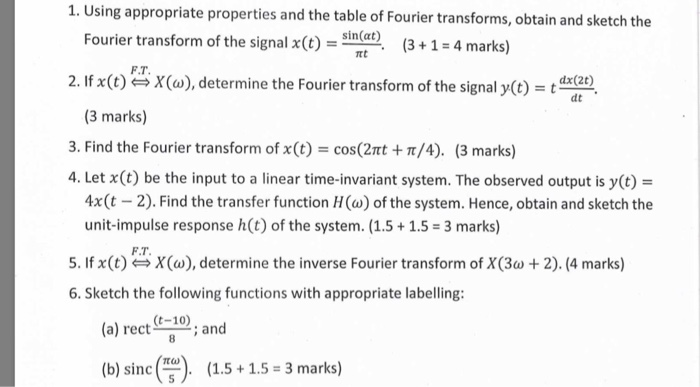1. Using appropriate properties and the table of Fourier transforms, obtain and sketch the sin(at) Fourier transform of the signal x()cn(31-4 marks) 2fX(a), determine the Fourier transform of the signal y(t)dx( F.T. dx(2t) dt (3 marks) 3. Find the Fourier transform of x(t)-cos(2t/4). (3 marks) 4. Let x(t) be the input to a linear time-invariant system. The observed output is y(t) 4x(t 2). Find the transfer function H() of the system. Hence, obtain and sketch the unit-impulse response h(t) of...

• ### QUESTION 3 (a) If the Fourier transform of (t) is X(c) = 12 (a+2)j@+6) determine the...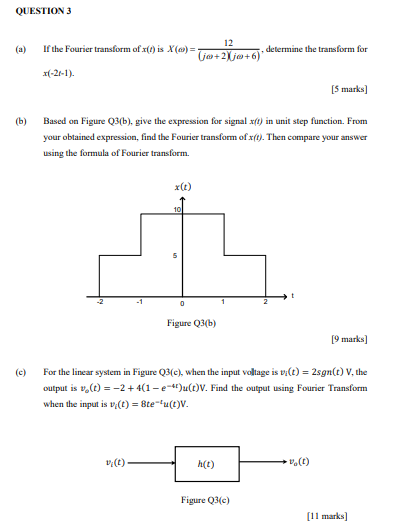QUESTION 3 (a) If the Fourier transform of (t) is X(c) = 12 (a+2)j@+6) determine the transform for (-21-1). [5 marks] (b) Based on Figure Q3(b), give the expression for signal xt) in unit step function. From your obtained expression, find the Fourier transform of x(). Then compare your answer using the formula of Fourier transform. x() 10 0 Figure Q3(b) [9 marks] 다. For the linear system in Figure Q3(e), when the input voltage is vr(t) = 2sgn(t) V....

• ### For a continuous time linear time-invariant system, the input-output relation is the following (x(t) the input, y(t) the...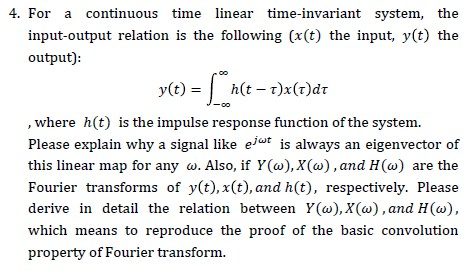For a continuous time linear time-invariant system, the input-output relation is the following (x(t) the input, y(t) the output): , where h(t) is the impulse response function of the system. Please explain why a signal like e/“* is always an eigenvector of this linear map for any w. Also, if ¥(w),X(w),and H(w) are the Fourier transforms of y(t),x(t),and h(t), respectively. Please derive in detail the relation between Y(w),X(w),and H(w), which means to reproduce the proof of the basic convolution property...

• ### h(n) is a stable system, , a = 50, Find the Z-transform H(n) Find the Fourier...

h(n) is a stable system, , a = 50, Find the Z-transform H(n) Find the Fourier Transform X(n) Using MATLAB, plot the frequency response from 0 to pi and from 0 to 2pi

• ### Explain how fourier transform is done? 1. (8 points) Suppose a particular discrete-time linear and time-invariant...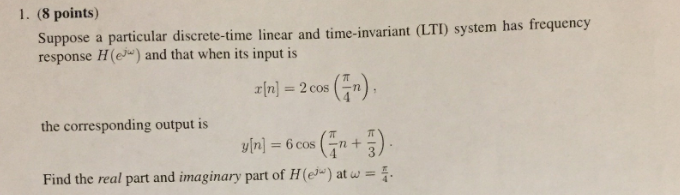Explain how fourier transform is done? 1. (8 points) Suppose a particular discrete-time linear and time-invariant (LTI) system has frequency response H(e) and that when its input is z[n] = 2 cos (n). the corresponding output is y[n] = 6 cos (n+ ) Find the real part and imaginary part of H(e)") at w = .

• ### 5. Fourier Transform and System Response (12 pts) A signal æ(t) = (e-t-e-3t)u(t) is input to...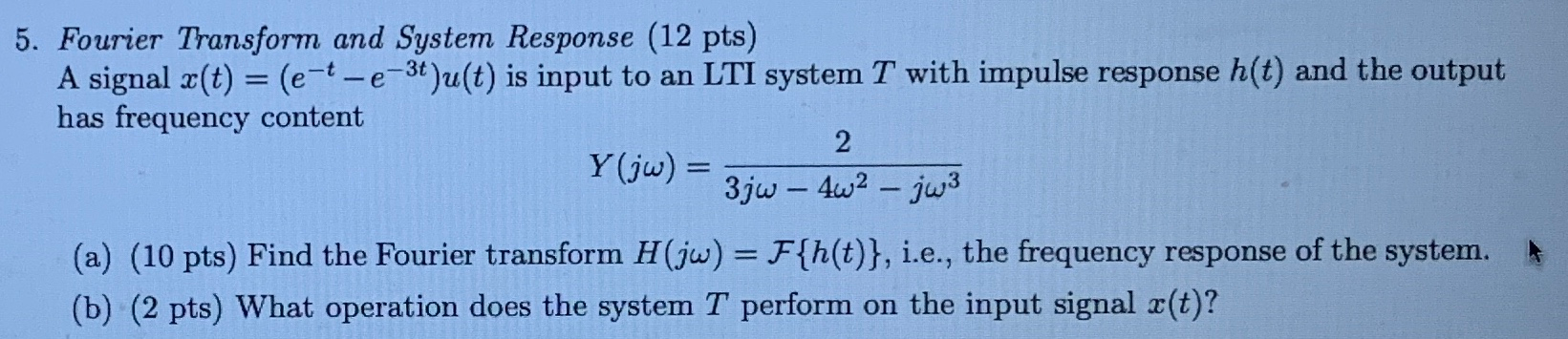5. Fourier Transform and System Response (12 pts) A signal æ(t) = (e-t-e-3t)u(t) is input to an LTI system T with impulse response h(t) and the output has frequency content Y(jw) = 3;w – 4w2 - jw3 (a) (10 pts) Find the Fourier transform H(jw) = F{h(t)}, i.e., the frequency response of the system. (b) (2 pts) What operation does the system T perform on the input signal x(t)?

• ### [b] State and prove frequency shifting property of Fourier transform Also find the fourier transform of...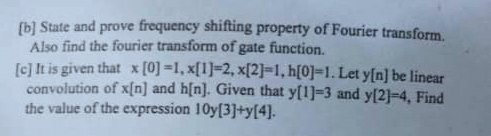[b] State and prove frequency shifting property of Fourier transform Also find the fourier transform of gate function. [c] It is given that x =1, x=2, x=1, h=1. Let y[n] be linear convolution of x[n) and h[n]. Given that y=3 and y-4. Find the value of the expression 10y+y.

• ### Problem 2: Consider an LTI system with input sequence x[n] and output sequence y[n] that satisfy...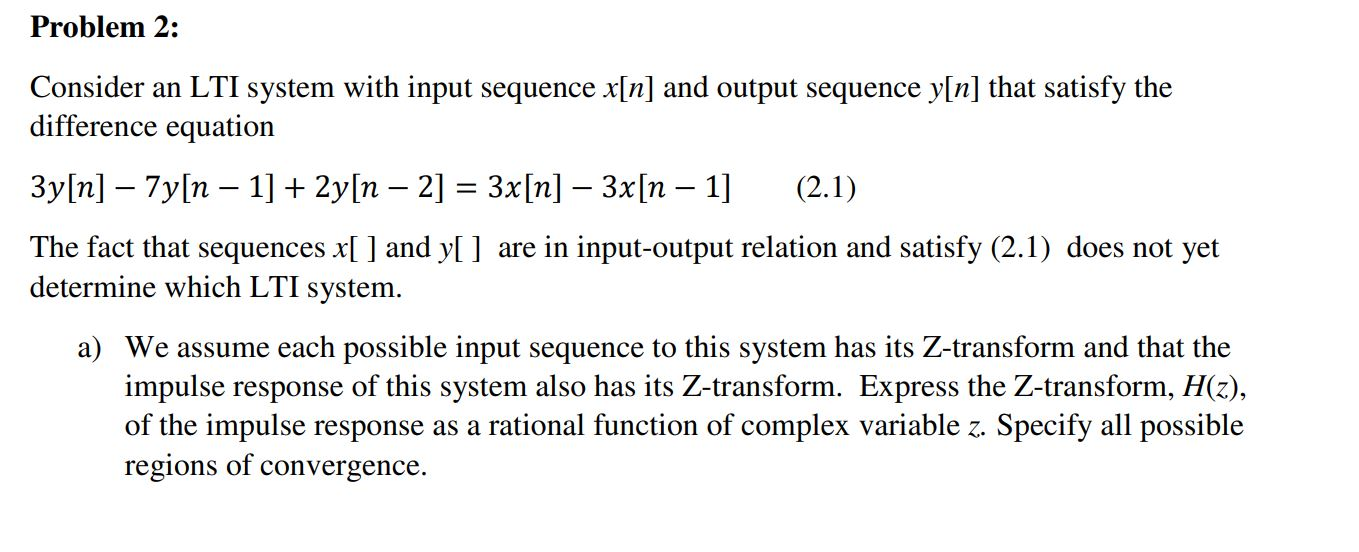Problem 2: Consider an LTI system with input sequence x[n] and output sequence y[n] that satisfy the difference equation 3y[n] – 7y[n – 1] + 2y[n – 2] = 3x[n] – 3x[n – 1] (2.1) The fact that sequences x[ ] and y[ ] are in input-output relation and satisfy (2.1) does not yet determine which LTI system. a) We assume each possible input sequence to this system has its Z-transform and that the impulse response of this system also...

• ### Question given an LTI system, characterized by the differential equation d’y() + 3 dy + 2y(t)...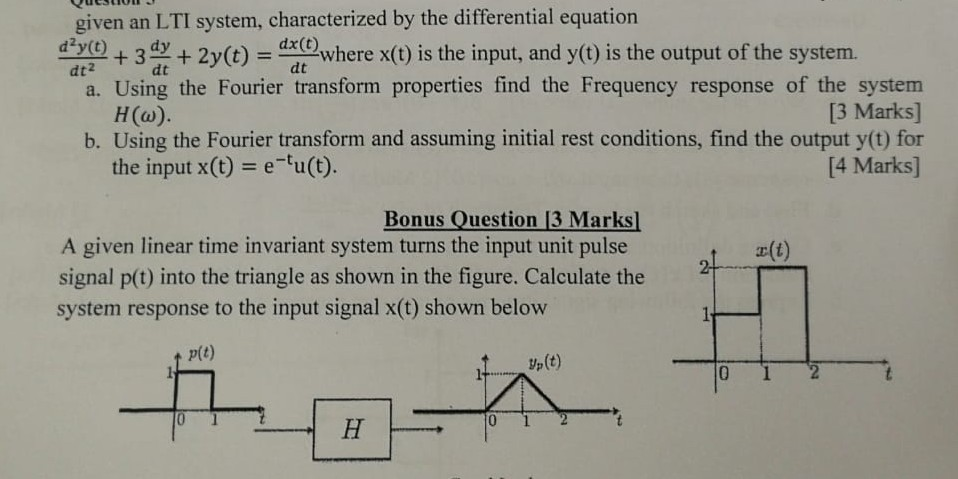Question given an LTI system, characterized by the differential equation d’y() + 3 dy + 2y(t) = dr where x(t) is the input, and y(t) is the output of the system. a. Using the Fourier transform properties find the Frequency response of the system Hw). [3 Marks] b. Using the Fourier transform and assuming initial rest conditions, find the output y(t) for the input x(t) = e-u(t). [4 Marks] Bonus Question 3 Marks A given linear time invariant system turns...

• ### Problem 3 Use tables of Fourier Transforms and properties to help deter- mine the Fourier transform...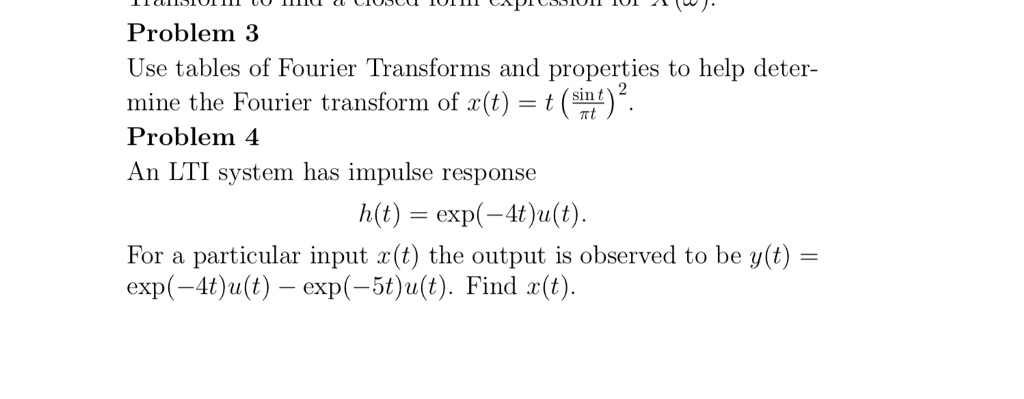Problem 3 Use tables of Fourier Transforms and properties to help deter- mine the Fourier transform of (t)t (sint Problem 4 An LTI system has impulse response )2 h(t) = exp(-4t)2(t) For a particular input (t) the output is observed to be y(t) exp(-4t)ult) exp(-5t)ult). Find ()